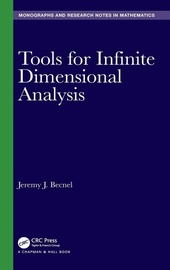# Tools for Infinite Dimensional Analysis

CRC Press
• 1. Auflage
• |
• erscheint ca. am 21. Dezember 2020

• Buch
• |
• Hardcover
• |
• 288 Seiten
978-0-367-54366-2 (ISBN)

Over the past six decades, several extremely important fields in mathematics have been developed. Among these are Itô calculus, Gaussian measures on Banach spaces, Malliavan calculus, and white noise distribution theory. These subjects have many applications, ranging from finance and economics to physics and biology.
Unfortunately, the background information required to conduct research in these subjects presents a tremendous roadblock. The background material primarily stems from an abstract subject known as infinite dimensional topological vector spaces. While this information forms the backdrop for these subjects, the books and papers written about topological vector spaces were never truly written for researchers studying infinite dimensional analysis. Thus, the literature for topological vector spaces is dense and difficult to digest, much of it being written prior to the 1960s.
Tools for Infinite Dimensional Analysis aims to address these problems by providing an introduction to the background material for infinite dimensional analysis that is friendly in style and accessible to graduate students and researchers studying the above-mentioned subjects. It will save current and future researchers countless hours and promote research in these areas by removing an obstacle in the path to beginning study in areas of infinite dimensional analysis.
Features

Focused approach to the subject matter

Suitable for graduate students as well as researchers

Detailed proofs of primary results
 Reihe: Sprache: Englisch Verlagsort: London | Großbritannien Verlagsgruppe: Taylor & Francis Ltd Zielgruppe: Für höhere Schule und Studium Maße: Höhe: 235 mm | Breite: 159 mm Schlagworte: ISBN-13: 978-0-367-54366-2 (9780367543662)weitere Ausgaben werden ermittelt
Jeremy J. Becnel was born in the rural community of Kraemer, Louisiana, in 1979 and completed his undergraduate studies in mathematics and computer science at Nicholls State University in May 2001. He earned a Master of Science degree from Louisiana State University in December 2002 and a PhD in August 2006. Immediately after graduation, Dr. Becnel began working at Stephen F. Austin State University (SFASU). He has worked at SFASU for fifteen years as a professor in the Department of Mathematics and the Department of Computer Science. During this time, Dr. Becnel has supervised numerous undergraduate research projects and graduate theses. He is a recipient of the National Science Foundation Division of Undergraduate Research grant and a Young Investigator's Grant from the National Security Agency, among others. Through the former grant, he has worked with colleagues to create CalcVR, a virtual reality app for multivariable calculus. His research interests include quantum computing, Radon transforms, probability theory, white noise analysis, and topological vector spaces. Dr. Becnel currently lives in the small town of Nacogdoches, Texas with his wife, Kesha, along with their daughters, Alyssa and Anna.
1. Schwartz Space. 1.1. The Schwartz Space. 1.2. The Schwartz Norm Topology. 1.3. Fourier Transform. 1.4. Physical Context. 1.5. Orthonormal Basis, Creation, and Annihilation Operators in L²(R). 1.6. Number Operator and the Spaces Sp(R). 1.7. The Projective Limit Topology on S(R). 1.8. L² norms on S(R). 1.9. Equivalence of the Topologies. 1.10. The Sequence Space. 1.11. Multidimensional. 2. Nuclear Spaces. 2.1. Topological Vector Spaces. 2.2. Countably-Normed Spaces. 2.3. Nuclear Space Structure. 2.4. Nuclear Space Construction from a Hilbert Space and an Operator. 3. Dual Space. 3.1. Linear Functionals. 3.2. Dual Space. 3.3. The Dual of a Nuclear Space. 3.4. Topologies on H'. 3.5. Reflexivity. 3.6. Convergence and Completeness in H'. 3.7. Properties of the Topology on H' and H_p. 3.8. Inductive Limit Topology. 3.9. Sequential Space. 3.10. First Countability (Lack Thereof). 3.11. Summary. 3.12. Abstract Kernel Theorem. 3.13. White Noise Dual. 3.14. Schwartz Space Dual. 3.15. Boxes and Continuous Functions. 4. Probability Theory. 4.1. Probability Theory Fundamentals. 4.2. Product Sigma Algebras in Infinite Dimensions. 4.3. Sigma Algebras on the Dual of a Nuclear Space. 4.4. Probability Measures on Infinite Dimensional Spaces. Appendix:Banach and Hilbert Spaces.
Schweitzer Klassifikation
• Mathematik
•Analysis
•Funktionalanalysis
• Mathematik
•Topologie
Thema Klassifikation
DNB DDC Sachgruppen
Dewey Decimal Classfication (DDC)
• Science
•Mathematics
•Analysis
BIC Classifikation
• Mathematics & science
•Mathematics
•Calculus & mathematical analysis
•Calculus
• Mathematics & science
•Mathematics
•Topology
BISAC Classifikation
• Mathematics
•Calculus
• Mathematics
•Topology

Noch nicht erschienen

 165,38 € inkl. 5% MwSt.